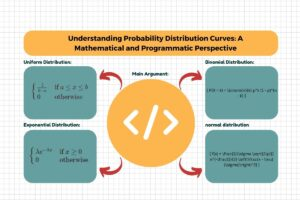### Understanding Probability Distribution Curves: A Mathematical and Programmatic Perspective

Introduction Probability distribution curves are fundamental concepts in statistics, providing insights into the likelihood of different outcomes in a random process. They serve as powerful